How Cheenta works to ensure student success?
Explore the Back-Story

# Test of Mathematics Solution Subjective 70 - Equal RootsThis is a Test of Mathematics Solution Subjective 70 (from ISI Entrance). The book, Test of Mathematics at 10+2 Level is Published by East West Press. This problem book is indispensable for the preparation of I.S.I. B.Stat and B.Math Entrance.

Also visit: I.S.I. & C.M.I. Entrance Course of Cheenta

## Problem

Suppose that all roots of the polynomial equation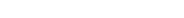= 0 are positive real numbers.
Show that all the roots of the polynomial are equal.

## Solution= 0
If the roots are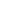,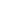,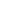and.
then,,and= 1
&+++= 4.
Now all of,,andare positive so AM-GM inequality is applicable.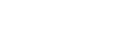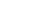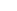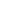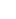1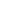1
Now we know equality in AM-GM occours if all the numbers are equal.So,,andare all equal.This is a Test of Mathematics Solution Subjective 70 (from ISI Entrance). The book, Test of Mathematics at 10+2 Level is Published by East West Press. This problem book is indispensable for the preparation of I.S.I. B.Stat and B.Math Entrance.

Also visit: I.S.I. & C.M.I. Entrance Course of Cheenta

## Problem

Suppose that all roots of the polynomial equation= 0 are positive real numbers.
Show that all the roots of the polynomial are equal.

## Solution= 0
If the roots are,,and.
then,,and= 1
&+++= 4.
Now all of,,andare positive so AM-GM inequality is applicable.11
Now we know equality in AM-GM occours if all the numbers are equal.So,,andare all equal.

### Knowledge Partner